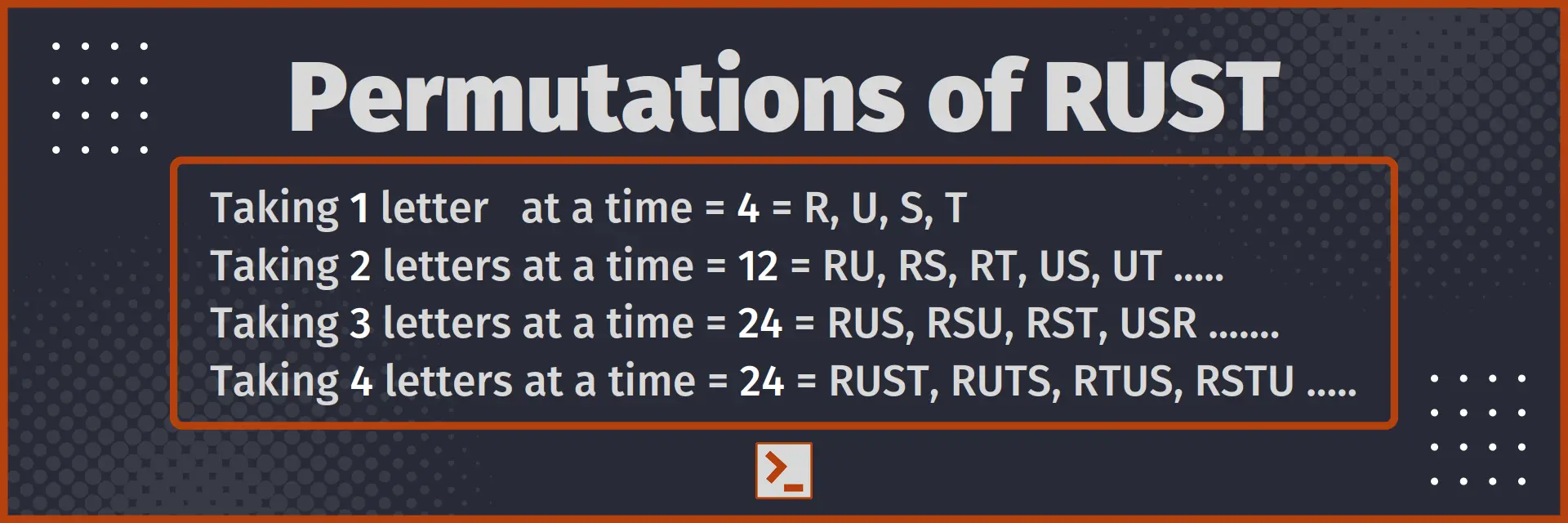# Permutation

and program to find the Permutation in Rust

## What is Permutation

Permutation is the number of ways in which some of the objects from a given set can be chosen and arranged. In permutation, the order in which things are arranged also matters, unlike in combination.

For example : Permutation of word RUST are RSTU, RSUT, RTSU, RTUS ..... 24 ways

If you want to read more about what Permutation means, I would recommend you to read from any High School Mathematics book of your preference.

In this article, we will use standard reference : the number of total objects to be arranged are denoted by n and the number of items chosen at a time are denoted by r.

So, total number of ways of arranging n unique items taking r at a time is written nPr. It will also be written as P(n, r)## Permutation formulae

### When all Objects are distinct

1. When repetition is allowed : If Repetition of an object is allowed, then we can simply write number of permutations = nr. We can calculate it using Exponent or Power function.

2. When repetition is not allowed : If Repetition of an object is not allowed, then permutations can be written as nPr = n! / (n-r)! We can calculate it using Factorial function

### When some objects are identical

1. When repetition is allowed : If Repetition of an object is allowed, then we can simply write number of permutations = kr, where k are total number of unique objects. We can calculate it using Exponent or Power function.
2. When repetition is not allowed : If repetition is not allowed, but there are n1 identical objects of type 1, n2 objects of type 2 ... nk objects of type k, then total permutations of all objects are
n! / n1! × n2! × ... nk!

## Program to find Permutation

Now, let us write a program in Rust Language to find the number of permutations when we are given n distinct objects, and we can arrange r at a time.

`fn permutation(n: i128, r: i128) -> i128{    // nPr = n! / (n-r)!    return factorial(n)/factorial(n-r);}`

With driver code

```fn permutation(n: i128, r: i128) -> i128{    // nPr = n! / (n-r)!    return factorial(n)/factorial(n-r);}
// Driver code
use std::io::stdin;fn take_int() -> i128 {    let mut input = String::new();    stdin().read_line(&mut input).unwrap();    return input.trim().parse().unwrap();}fn factorial(number : i128) -> i128{    let mut factorial : i128 = 1;    for i in 1..(number+1) { factorial*=i; }    return factorial;}
pub fn main() {    let n = take_int();    let r = take_int();    println!("{}", permutation(n, r));}```

Input

6
4

Output

360

Time Complexity : O( n )
Space Complexity : O( 1 )

## Conclusion

Permutation is the number of ways in which some of the objects from a given set can be chosen and arranged. In this article, we saw the formulae for calculating number of permutation for given n and r and also made a program to find number of permutations in Rust.

Here is the function for easy access

```fn factorial(number : i128) -> i128{    let mut factorial : i128 = 1;    for i in 1..(number+1) { factorial*=i; }    return factorial;}
fn permutation(n: i128, r: i128) -> i128{    return factorial(n)/factorial(n-r);}```

Thank You